# remainder

(redirected from remaindering)
Also found in: Dictionary, Thesaurus, Legal, Financial.

## remainder

1. Maths
a. the amount left over when one quantity cannot be exactly divided by another
b. another name for difference
2. Property law a future interest in property; an interest in a particular estate that will pass to one at some future date, as on the death of the current possessor

## Remainder

The remainder in an approximation formula is the difference between the exact and the approximate values of the expression represented by the formula. A remainder can take different forms depending on the nature of the approximation formula. The task of investigating a remainder usually consists in obtaining estimates for it. For example, corresponding to the approximate formula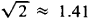we have the exact equality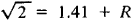where the expression R is the remainder for the approximation 1.41 for the number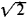and it is known that 0.004 < R < 0.005.

Remainders are constantly encountered in asymptotic formulas. For example, for the number π (x) of primes not exceeding x we have the asymptotic formula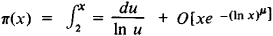where μ is any positive number less than 3/5. Here, the remainder, which is the difference between the functions π (x) an ∫x2 du/ln u for x2, is written in the form

O[xe-(In x)μ

where the letter O indicates that the remainder does not exceed the expression

Cxe-(In x)μ

in absolute value, C being some positive constant. Remainders are found in formulas that give approximate representations of functions. For example, in the Taylor formula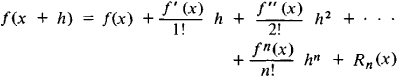the remainder Rn (x) in Lagrange’s form is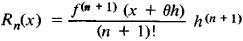where θ is a number such that 0 < θ < 1; θ generally depends on the values of x and h. The presence of 0 in the formula for Rn(x) introduces an element of indefiniteness; such indefinite-ness is inherent in many formulas for the remainder.

Remainders also occur in quadrature formulas and interpolation formulas.

## remainder

[ri′mān·dər]
(mathematics)
The remaining integer when a division of an integer by another is performed; if l = m · p + r, where l, m, p, and r are integers and r is less than p, then r is the remainder when l is divided by p.
The remaining polynomial when division of a polynomial is performed; if l = m · p + r, where l, m, p, and r are polynomials, and the degree of r is less than that of p, then r is the remainder when l is divided by p.
The remaining part of a convergent infinite series after a computation, for some n, of the first n terms.

## remainder

An interest in property that confers a right to possession in someone other than the grantor or his heirs upon the termination of a prior interest, such as following the death of a life tenant.
Site: Follow: Share:
Open / Close